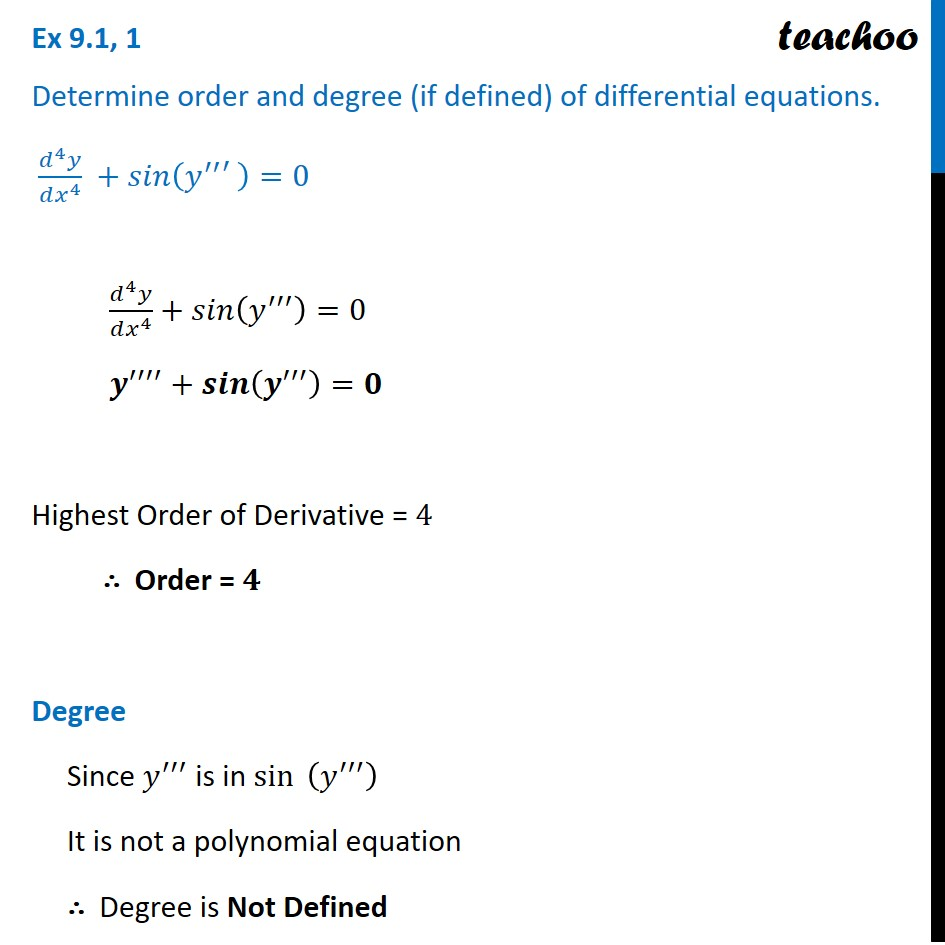Ex 9.1

Chapter 9 Class 12 Differential Equations
Serial order wiseLearn in your speed, with individual attention - Teachoo Maths 1-on-1 Class

### Transcript

Ex 9.1, 1 Determine order and degree (if defined) of differential equations. (𝑑^4 𝑦)/(𝑑𝑥^4 ) +𝑠𝑖𝑛(𝑦^′′′ )=0 (𝑑^4 𝑦)/(𝑑𝑥^4 )+𝑠𝑖𝑛(𝑦^′′′ )=0 𝒚^′′′′+𝒔𝒊𝒏(𝒚^′′′ )=𝟎 Highest Order of Derivative = 4 ∴ Order = 𝟒 Degree Since 𝑦^′′′ is in sin⁡〖 (𝑦^′′′ )〗 It is not a polynomial equation ∴ Degree is Not Defined